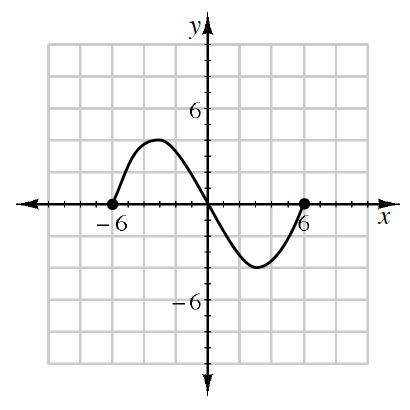### Home > CCAA8 > Chapter 1 Unit 1 > Lesson CCA: 1.2.5 > Problem1-81

1-81.

Use the relationship graphed at right to answer the questions below.

1. Is the relation a function?

Does there appear to be more than one $y$-value for each value of $x$?

Yes, it is a function.

1. What is the domain?

Look at the $x$-intercepts on the graph.

$−6\le x\le6$

1. What is the range?

Look at the lowest and highest points on the graph.

$−4\le y\le4$